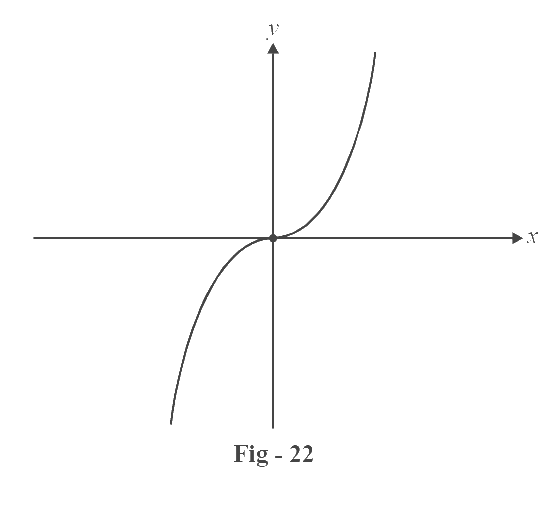# Domains and Ranges of Standard Functions Set 1

Go back to  'Functions'

Listed below are some functions we’ll encounter frequently. Note carefully their domains and ranges and how they have been plotted.

1. Constant function $$f\,(x) = k$$

Domain = $$\mathbb{R}$$

Range = $$\{ k\}$$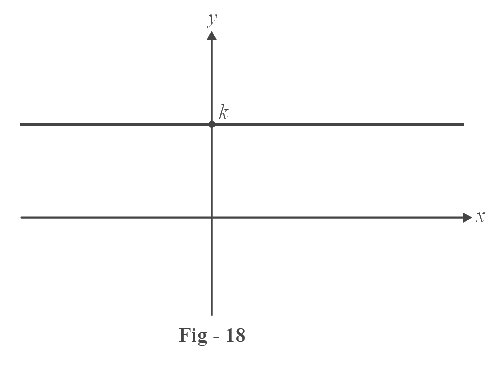2. Identity function $$f\,(x) = x$$

Domain = $$\mathbb{R}$$

Range = $$\mathbb{R}$$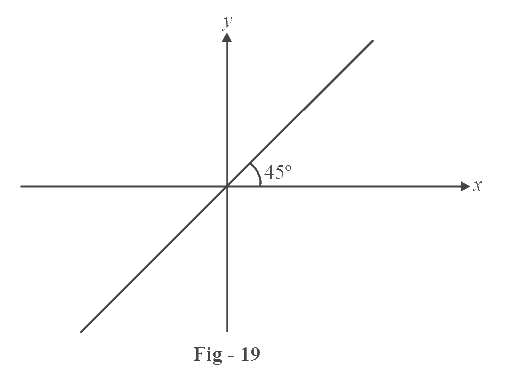3. Linear function $$f\,(x) = mx + c$$

Domain = $$\mathbb{R}$$

Range = $$\mathbb{R}$$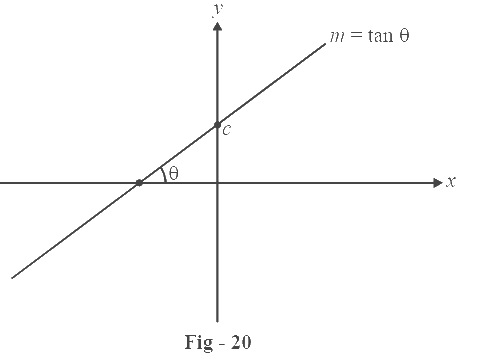4. Square function $$f\,(x) = {x^2}$$

Domain = $$\mathbb{R}$$

Range = [0, $$\infty$$)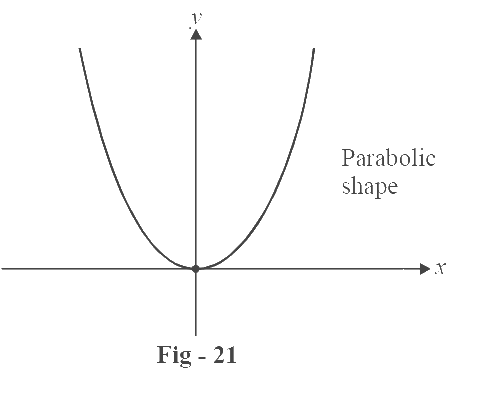5. Cube function $$f\,(x) = {x^3}$$

Domain = $$\mathbb{R}$$

Range = $$\mathbb{R}$$